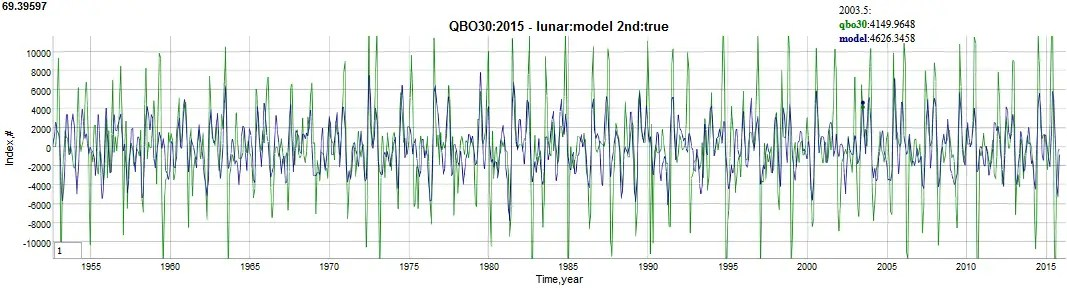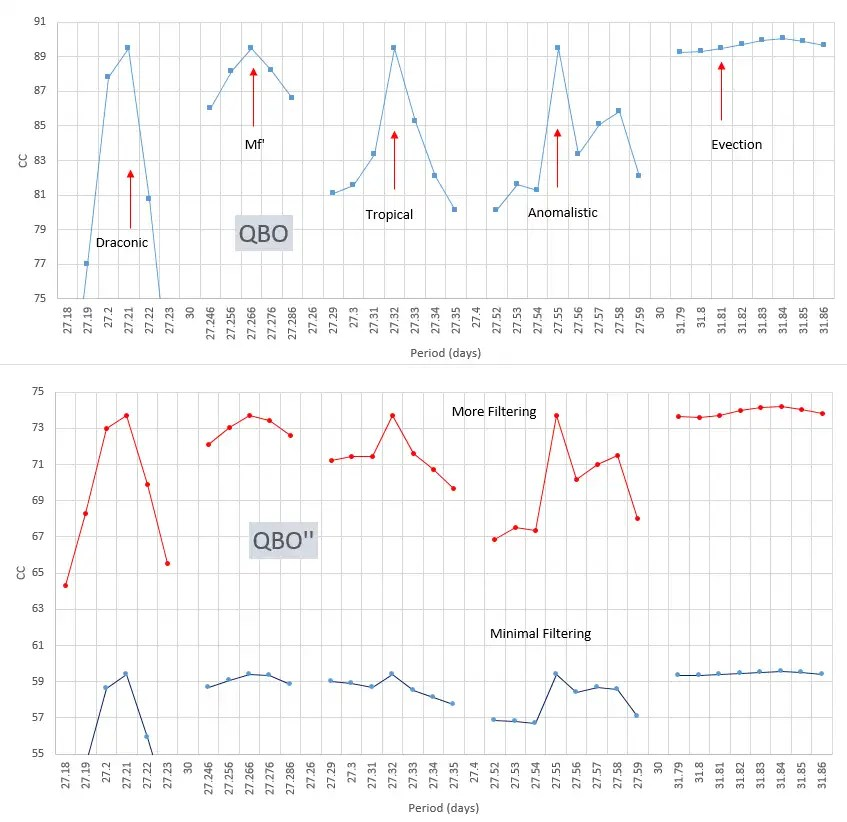# QBO Model Validation

[mathjax]The strongly periodic Quasi-Biennial Oscillation (QBO) of stratospheric winds is externally forced by a cyclic mechanism. This is obvious in spite of what the current scientific literature says about the nature of QBO;  the general consensus being that the QBO emerges as a complex natural resonant response of other atmospheric factors . Yet, an experienced experimental scientist should not consider that as the only plausible premise. It is in fact exceedingly rare for a phenomena of that global a scale to be the result of a natural resonance, and for the QBO to be a resonance is clearly an untenable hypothesis based on historical precedent. There simply aren’t any climate phenomena that behave similarly and are not reliant on lunar or solar periods.

What causes the oscillation then?

The obvious candidate for the QBO is a lunar forcing in the form of an atmospheric tide. Tides, as in the oceanic tides, result from the gravitational tug of the moon and sun (and other bodies). For atmospheric tides, the gravitational pull of the moon is sufficient to create pressure differentials — and in the case of QBO the pressure will set the stratospheric winds in motion, and then reverse course when the lunar pull ebbs.

So if it is so obvious, why has no one one postulated this mechanism?

Well, atmospheric scientists have in fact proposed lunar forcing as a candidate, but quickly dismissed this as a factor since the long-period lunar cycling is much faster than the ~28-29 month periodicity of the QBO.  However, upon closer examination a very narrow window of opportunity does exist for this factor to emerge.  It is narrow since the cycling periods for the  lunar potential have to match precisely the known periods of the QBO for the idea to be viable.   One very feasible way for this to happen is if the lunar period was reinforced by another cyclic factor such that the lunar month was extended to the ~28 month period observed (along with potentially other harmonics uncovered in the QBO).

As a subtly obvious mechanism, the reinforcement of the lunar cycle with the seasonal solar cycle can if fact produce a factor exceedingly close to the 28 month period of the QBO.  Mechanically this happens via a multiplying reinforcement of the 27.212 day Draconic or nodal lunar cycle with the yearly or seasonal/biennial solar signal.   The math behind this is straightforwardly described here, and results in a strong signal aliased at 2.366 years = 28.4 months.  Since this is a result of aliasing, other signals  should be found at frequency multiples of 2$$pi$$ leading to aliased harmonics at 0.703 and 0.413 years.

To validate that these are strong signals in QBO, one can take the second-derivative of the 30 hPa QBO time series, and feed that into the Eureqa symbolic regression machine learning tool.  After more than 10 trillion formula calculations, the solution is shown in Figure 1 below.Fig 1:  Eureqa results when applied to a QBO time-series 2nd derivative. The lowest error solution (shown in reverse highlighted blue) feature as strongest sinusoidal factors the three seasonally aliased Draconic harmonics.

Taking the second derivative is important because (1) it enhances the higher frequency factors that can occur as a result of the lunar forcing, and (2) is a key to the wave equation solution of any forced response.  And sure enough, what Eureqa finds as the strongest factors are the three aliased Draconic factors predicted above.

In Figure 2 below the three strongest factors discovered align strongly with the predicted aliased Draconic periods. Having one of these factors align might be a coincidence, but discovering a strong simultaneous correlation with all three is highly significant in a statistical sense.Fig. 2 : Alignment of the three strongest sinusoidal periods found via machine learning with those predicted via seasonally reinforced Draconic lunar tidal forcing.

As a physical rationale, the Draconic or nodal pumping is likely the strongest mechanism for forcing of QBO.  As the moon’s gravitational pull can impact the northern or southern hemisphere to a greatest extent at the nodal extreme, this in conjunction with the timing of a seasonal hotspot in the same hemisphere is all that is required to create a forcing impetus for the QBO.  Combine that with the  reinforcement at a strict cycle,  we have the most plausible mechanism for QBO forcing.  In Figure 3 below, we can run a multiple regression analysis with respect to the main aliased lunar factors and find a remarkable fit to the observed QBO signal.  This is as good a quality fit to known factors as one can get with (for instance) the long-understood oceanic tide modeling.  Nearly every peak and trough is accounted for.Figure 3:  Fit of a combination of sinusoidal luni-solar tidal factors to the 2nd derivation of the 30 hPa QBO time series.

As for model robustness, the set of Draconic plus other aliased lunar factors (anomalistic, tropical,etc) are parametrically sensitive to changes from the exact period chosen.  As shown in Figure 4, even slight variations from the standard lunar periods cause a rapid reduction in model fit.Fig. 4:  Parametric sensitivity of lunar QBO model.

What can we conclude from this?

For this lunar forced model of the Quasi-Biennial Oscillation, an exact fit to theory is necessary to give it credibility.  A credentialed atmospheric scientist once said “it is unlikely that lunar periods could be produced by anything other than the lunar tidal potential”Guess who that was?

With the current interest in the experimental detection of the long-conjectured gravitational waves, it should come as no surprise that other discoveries relating to gravity will continue to happen.  In this case, here we have another phenomenon that is just as subtle, but hiding in plain sight. The validation of a luni-solar forced ENSO model is sure to come, given the equally surprising result that the Chandler wobble has lunar origins.

## References

 The key here is that general circulation models (GCM) of the earth’s atmosphere reproduce behavior of QBO, indicating that it is an emergent behavior not tied to a single mechanism.  This is an early cite: Takahashi, M., N. Zhao & T. Kumakura,1997: Equatorial waves in a General Circulation Model simulating a quasi-biennial oscillation. J. Meteor. Soc. Japan, 75,529-539.

## 4 thoughts on “QBO Model Validation”

1.admin

Here is a paper on teasing what appear to be non-linear oscillations out of the QBO.

 S. Pulkkinen, “Nonlinear kernel density principal component analysis with application to climate data,” Statistics and Computing, vol. 26, no. 1–2, pp. 471–492, 2016. pdf

Obviously the fit is good because once you start fiddling with arbitrarily shaped periodic components, you can match anything.

Like

2.admin

An interesting possibility for incrementally improving the excellent fit of the QBO is to consider a saturation function for the peaks and valleys. The QBO wind speeds give the impression that they limit out at a certain value. One way to model this is to apply a saturating factor such as

QBOs(t)= QBO(t) / (1+k|QBO(t)|)

This is essentially an application of a gradual diminishing returns factor so once the value in the denominator achieves a certain value it cancels the numerator. I realize that is a bit of a heuristic but the physics behind wind does show a limiting factor and this may be the simplest means to represent that behavior.

The improvement in fit is about 0.05 in the correlation coefficient.

Like